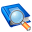# Glossary

A | B | C | D | E | F | G | H | I | J | K | L | M | N | O | P | Q | R | S | T | U | V | W | X | Y | Z

## K

### K-factor

In bending, the k-factor defines the location of the neutral axis in the material and is dependent upon several factors, such as the material, bending operation, bend angle, etc. The k-factor is calculated as the ratio of the distance of the neutral axis (measured from the inside bend surface) to the material thickness. Values for the k-factor are typically greater than 0.25 and cannot exceed 0.50.Neutral axisBending diagram